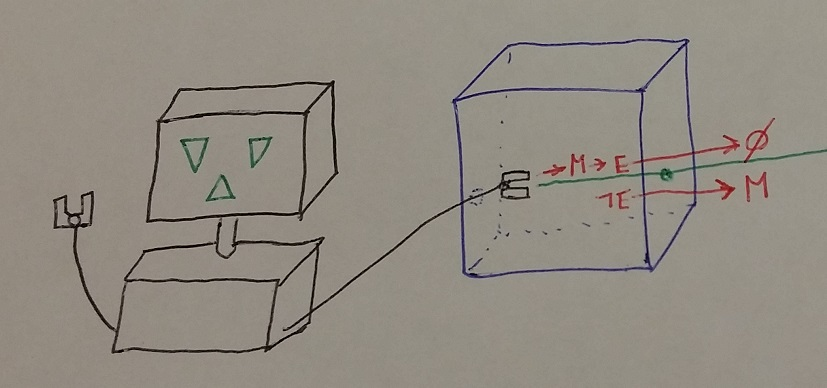# AI printing the utility value it’s maximising

A putative new idea for AI control; index here.

The utility function mentioned here is somewhat peculiar; see here.

Paul Christiano asked whether we could get an agent to print out the expectation of the utility that it was currently maximising. As I’m always eager to please, here’s a design to do that.

# The setup

The setup is slightly different to that presented previously. In particular, the agent is no longer boxed, though it has access to a box it can use to emit a message . As before, there is an erasure process that will erase the message (and will let it through unimpeded).Assume (I’ll be using the factor a lot in this example, for illustration, though all the different factors need not be the same, nor exactly set to this). Let be a utility function bounded between and .

The content of is a single number ; we’ll want this number to be the AI’s estimate of the utility of . More precisely, we want it to be the AI’s estimate of ’s expected utility, given -- ie given that the message is not read. Of particular importance is the fact that . Thus when the message is erased, its contents don’t matter.

Let be the index function for occurring (thus ). Then give the AI the utility function:

If the term wasn’t there, this would motivate the AI to output the that was equal to the expectation .

Let’s differentiate by , giving:

The first two terms are zero when . Around that point, the magnitude of this derivative is determined by the term, which is multiplied by , which, in expectation, is . By the assumption on the bounds of , is of magnitude at most . Therefore, the which maximises must be within of . If this is a reasonable tolerance, in the estimate, then will motivate the AI to output its estimate close to .

Then the AI will end up maximising , which means it will maximise . Thus the agent outputs an almost correct and also maximises the expectation of .

Why did I include the term, then, if all it does is mess up the output a little? That’s because will happen with probability, and, after that, we don’t want the AI left with a utility function that ignores the possibility of . One in a thousand (one in a million weight, a thousand to one prior) contrary odds seem to be odds that could be overcome by careful observation after happens, so the AI doesn’t have to end up acting as if it believed impossible things. If it turns out that the AI can’t overcome that deficit, then even better: that means the AI can’t easily distinguish from , so can’t be sure we know or not.

Note the tension here—reducing makes the estimate more accurate, but runs the risk of making the AI act as if it believed very odd things after is sent.

Note that before choosing , the AI is maximising an approximation of , while after choosing , it is maximising some multiple of .

# Multiple estimates

The method doesn’t generalise obviously to having the AI report multiple estimates of its expected utility (say a thousand or more), without having to make some the numbers so extreme that the AI could start to entertain `miraculous’ hypotheses. Ideally, we’d want to use proper corrigibility to reset the AI after each estimate.

# Robust, but imperfect and unsafe

The method is robust, in that it doesn’t matter if we’ve mis-estimated a bit (since it outputs , not ). Even if the setup is uncertain—say allows the message to be read once every thousand times—this just results in the error in being a bit larger, rather than the method collapsing.

The method is imperfect, as an AI would not behave exactly as a maximiser—and not just because of the error in . Specifically, the AI is motivated to discover much more about the expected value of that it would otherwise. Suppose, for instance, that pressing the red button would set while pressing the blue button would set to either or . A pure -maximiser would just press the blue button; but a -maximiser would also want to figure out what happened with after pressing the blue button.

The method isn’t safe—and not only because the AI motivated to get extra information about (and is unboxed). If it could prevent us from reading in the world, it would do so, if the cost to was trivial (which doesn’t mean the cost to us need be trivial).